# Streamlit 入门介绍

## 前言

`Streamlit` 是一个 Python Web 应用框架。但和常规 Web 框架，如 `Flask/Django` 的不同之处在于，它不需要你去编写任何客户端代码（HTML/CSS/JS），只需要编写普通的 `Python` 模块，就可以在很短的时间内创建美观并具备高度交互性的界面，从而快速生成数据分析或者机器学习的结果；另一方面，和那些只能通过拖拽生成的工具也不同的是，你仍然具有对代码的完整控制权。

## 安装与启动

`Streamlit` 是一个 `Python` 软件包，它同时支持 `Python` 2/3。当然，在现在这个时间，相信绝大多数人已经没有理由再去用 Py2 了。在平台支持方面，`Streamlit` 支持 `Windows/Mac/Linux` 三大操作系系统，但它主要是在 `Posix` 系统下开发的，在 `Windows` 上可能会遇到一些兼容性方面的问题，因此最好是在从 `Linux``Mac` 来运行。`Windows 10` 的用户建议使用虚拟机或 `WSL`

``````\$ pip install streamlit
``````

`Streamlit` 包自带了一个管理命令行。我们可以验证安装是否正常。在写作本文时，Streamlit 的版本是 `0.56`

``````\$ streamlit --version
Streamlit, version 0.56.0
``````

``````\$ streamlit hello
``````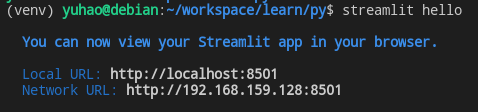• 左边是一个可折叠的区域，也就是 Sidebar（这是可选的）；
• 中间是主要内容的输出区域；
• 右上角是 `Streamlit` 的系统菜单，包含一些常用的命令和选项。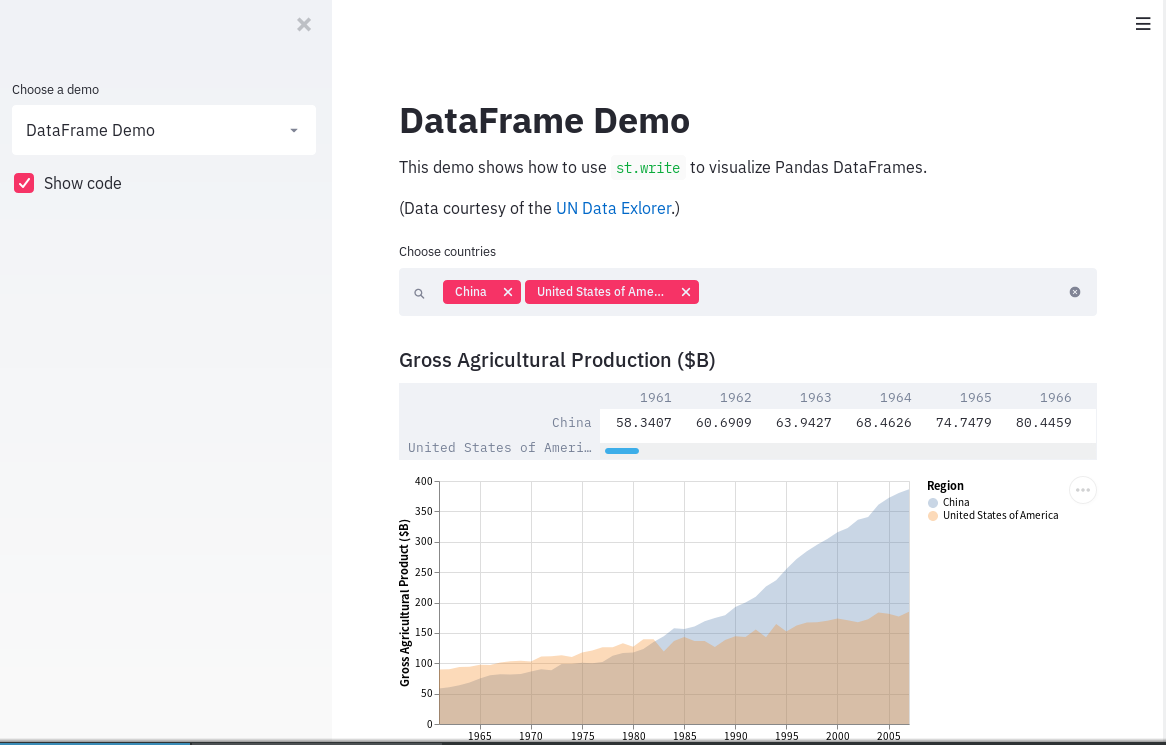## 基本示例

``````import streamlit as st

"Hello World"
``````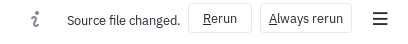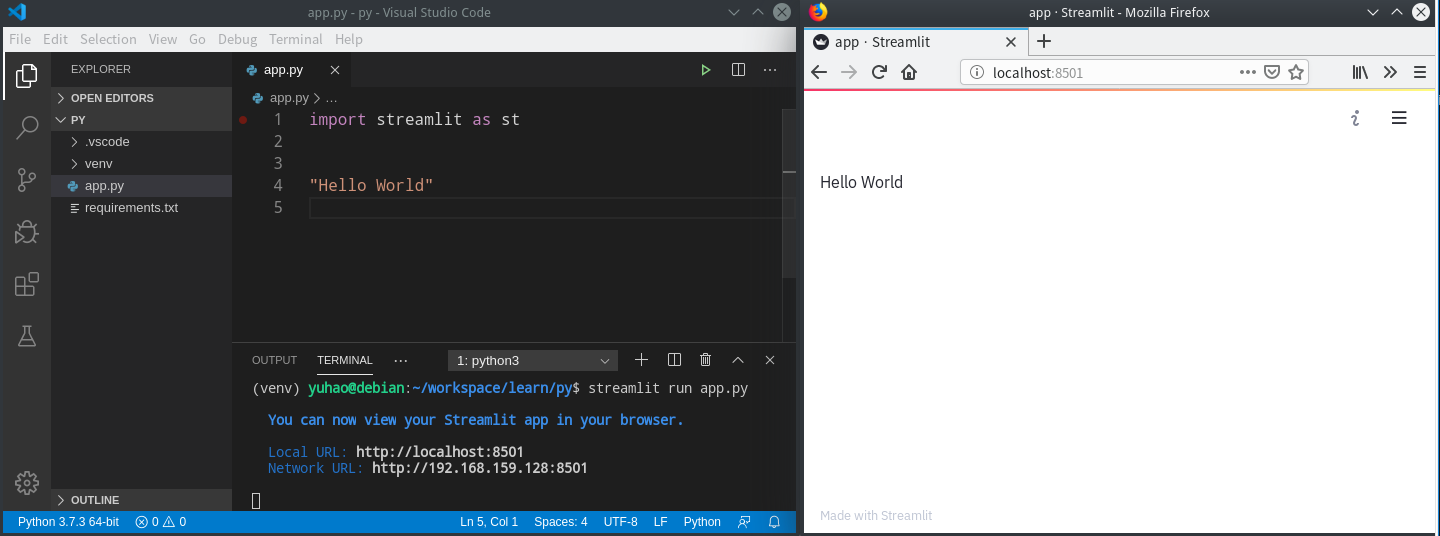``````import streamlit as st

st.write("Hello World")
``````

## 组件

### 文本组件

`Streamlit` 提供的文本组件比较简单，标题只有两级（`Header``SubHeader`）。对于大段的内容，纯文本形式可使用 `text`，复杂格式可使用 `Markdown`

``````import streamlit as st

st.text("Simple text")
st.markdown("""
- chapter 01
- chapter 02
- chapter 03

Emojis: :+1: :sunglasses:

From: [Github](https://github.com)""")
``````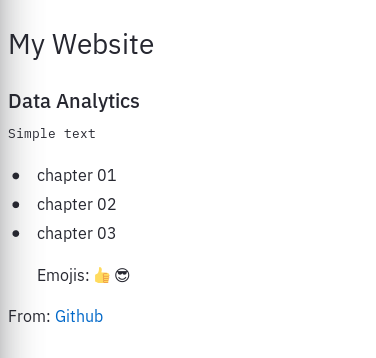`Streamlit` 也能够生成带风格样式的提示信息，例如：

``````import streamlit as st
import numpy as np
import pandas as pd
import altair as alt
import time
from datetime import datetime

st.success('Success!')
st.warning('Warning!')
st.error('Error!')
``````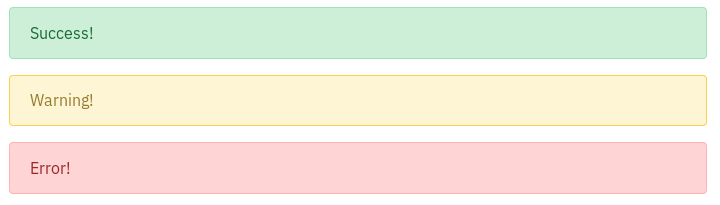`Streamlit` 还能显示程序代码（不过目前只支持 `Python`）：

``````st.code("""
class MyClass:
def __init__(self):
super(MyClass, self).__init__()
""")
``````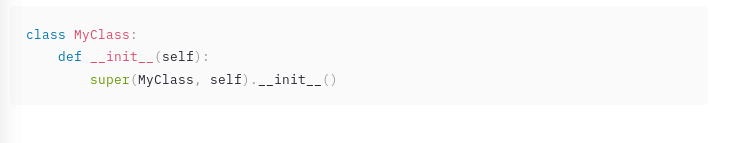``````st.json({
"pagination": {
"total": 100,
"per_page": 20,
"page": 1,
},
"items": [
{
"id": 1,
"is_superuser": True
}
]
})
``````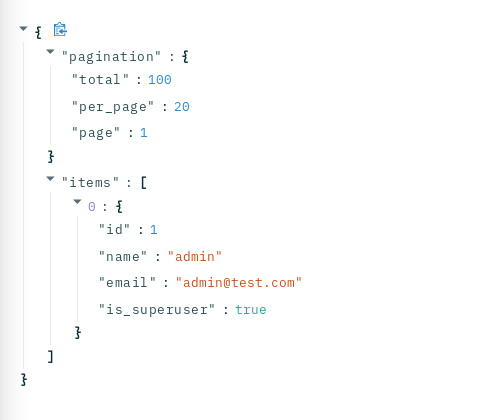``````with st.echo():
if st.button('Click Me'):
st.text('Button clicked!')
``````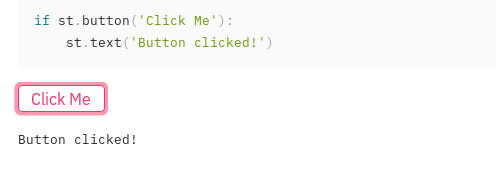### 数据表格

`Streamlit` 支持两种表格形式：可重新排序的动态样式表格，以及静态的纯 HTML 表格。它们分别用 `dataframe()``table()` 方法创建。它们的数据源通常是 `Pandas DataFrame`，也可以是 `Python``Numpy` 多维数组。

``````import streamlit as st
import numpy as np
import pandas as pd

df = pd.DataFrame(
np.random.randn(10, 20),
columns=('列 %d' % (i+1) for i in range(20)))

st.dataframe(df)
st.table(df)
``````

``````st.dataframe(df.style.highlight_max(axis=0))
``````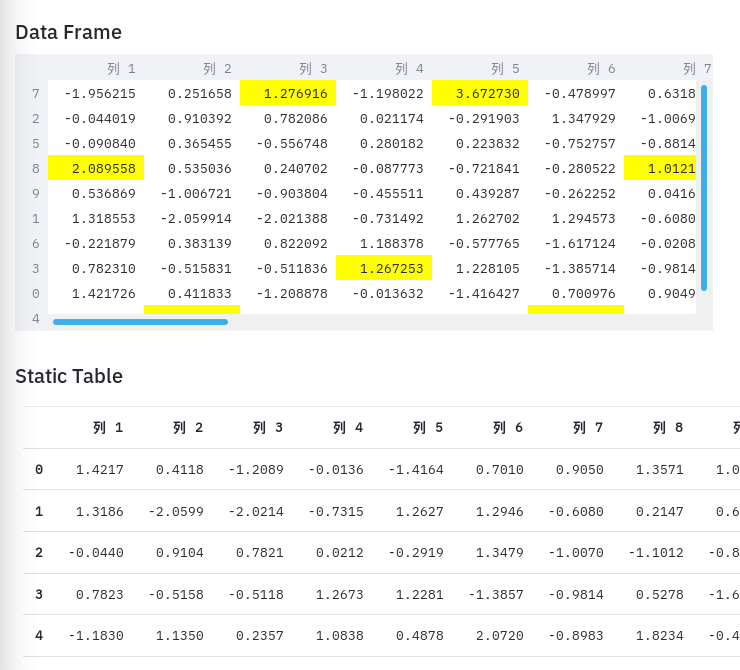### 图表与地图

`Streamlit` 支持多种图表库和图表类型。具体支持哪些图表取决于底层所使用的图表库，`Streamlit` 只是对它们进行了轻量级的包装。如果不明确指定的话，默认接口使用 `Matplotlib`

``````import streamlit as st
import numpy as np
import pandas as pd

chart_data = pd.DataFrame(
np.random.randn(20, 3),
columns=['Serie 1', 'Seria 2', 'Seria 3'])

st.line_chart(chart_data)
``````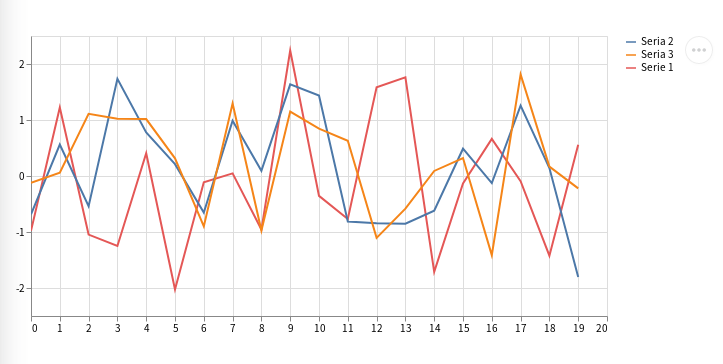``````import streamlit as st
import numpy as np
import pandas as pd
import altair as alt

df = pd.DataFrame(np.random.randn(200, 3),
columns=['a', 'b', 'c'])
c = alt.Chart(df).mark_circle().encode(
x='a', y='b', size='c', color='c')
st.altair_chart(c)
``````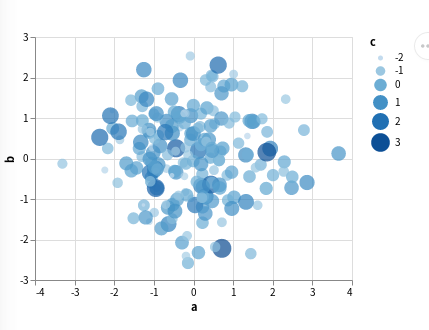``````df = pd.DataFrame(
np.random.randn(100, 2) / [50, 50] + [22.56, 114.03],
columns=['lat', 'lon'])
st.map(df)
``````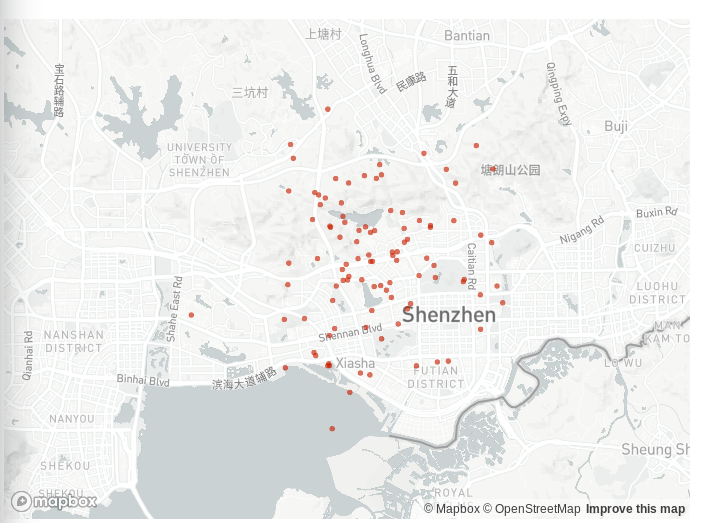### 交互组件

``````import streamlit as st

x = st.slider('Select a value between 0-100')
st.write(x, 'squared is', x * x)
``````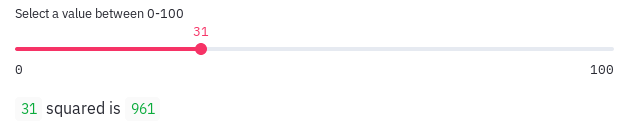``````import streamlit as st
import time

value = 0
progress = st.progress(value)
label = st.empty()
for i in range(0, 100):
value += 1
progress.progress(value)
label.text(f"Running progress: {value}%")
time.sleep(0.2)
label.text("Running completed.")
``````

``````import streamlit as st

label = st.empty()
label.warning('Running in progress...')
time.sleep(2)
label.success('Running finished.')
``````

`Streamlit` 也包括常用的输入组件。最简单的组件是按钮，但是它的接口可能有点不太直观：

``````import streamlit as st

if st.button('Click me'):
st.text('Button clicked!')
``````

st.button() 生成了一个按钮实例，但它返回的不是某种 Button 对象，而是一个 bool 标志，表示该按钮有没有被点击。虽然用法很简单，但有点不太符合直觉，所以需要注意写法。``````import streamlit as st

('Comedy', 'Drama', 'Documentary'))
st.write(f"You selected {genre}")

option = st.selectbox(
'How would you like to be contacted?',
('Email', 'Home phone', 'Mobile phone'))
st.write(f"You selected {option}")
``````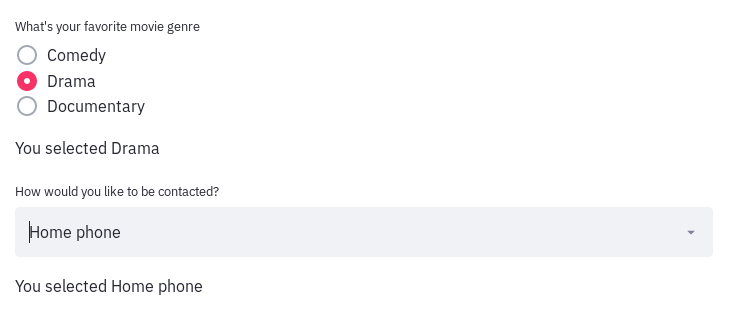### 边栏

``````import streamlit as st
import numpy as np
import pandas as pd
import altair as alt

chart_type = st.sidebar.selectbox(
'Which chart are you perfer?',
('Matplotlib', 'Altair')
)

chart_data = pd.DataFrame(
np.random.randn(20, 3),
columns=['a', 'b', 'c'])
if chart_type == 'Matplotlib':
st.line_chart(chart_data)
elif chart_type == 'Altair':
c = alt.Chart(chart_data).mark_circle().encode(
x='a', y='b', size='c', color='c')
st.altair_chart(c)
``````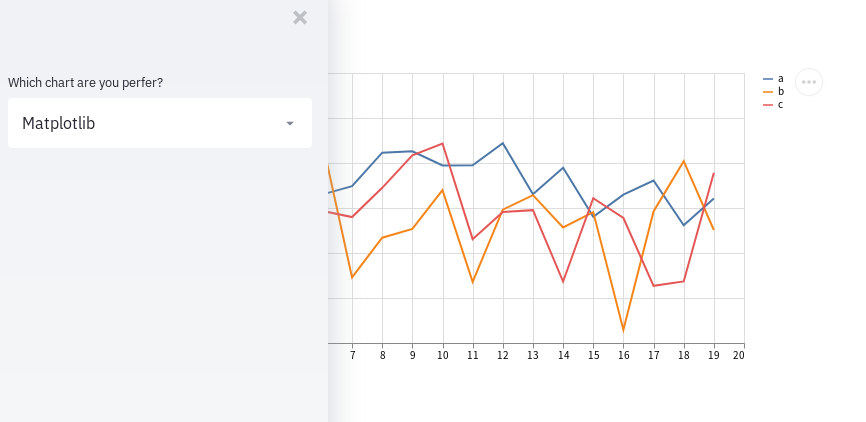## 配置

`Streamlit` 有很多配置项可以用来控制它在运行时的行为，它们大都是有默认值的，因此我们在最初的开发阶段可以不用关心，不过在生产环境有些可能就要调整了。比如在运行程序的时候我们注意到了，默认的服务端口是 8501，这个端口是否可以变化？当然可以通过配置来修改。

``````streamlit config show
``````### 方法一：通过全局配置

``````[server]
port = 8000
``````

### 方法二：通过环境变量

``````STREAMLIT_SERVER_PORT=8000 streamlit run app.py
``````

### 方法三：通过命令行参数

``````streamlit run app.py --server.port 8000
``````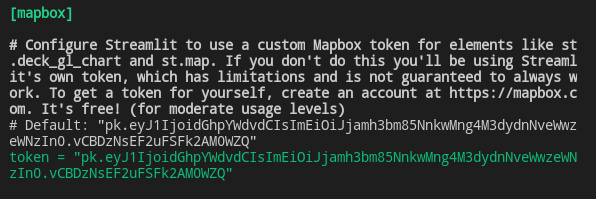## 运行机制与缓存

`Streamlit` 为提供缓存的机制是：将耗时但不需要频繁重新计算的操作封装为一个函数，并用 `cache` 装饰器来标识。我们看一个简单的示例：

``````import streamlit as st
from datetime import datetime

@st.cache
def calc(a, b):
time.sleep(2)
return a * b

start = datetime.now()
a, b = 10, 20
result = calc(a, b)
elapsed = (datetime.now() - start).total_seconds()
st.write(f"{a}*{b}={result}, Elapsed: {elapsed}")
``````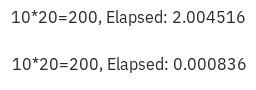• 传入的参数
• 函数引用到的其他变量
• 函数方法体
• 函数所引用的其他函数

## 部署

``````server {
listen 80;
listen [::]:80;
server_name         mysite.com;
return 301 https://\$host\$request_uri;
}

server {
listen 443 ssl;
server_name         mysite.com;
ssl_certificate     /etc/letsencrypt/live/mysite.com/fullchain.pem;
ssl_certificate_key /etc/letsencrypt/live/mysite.com/privkey.pem;

location ~ /\.ht {
deny all;
}

location / {
proxy_pass          http://127.0.0.1:8051;
}
}
``````

## 示例与资源

• 官网
• 文档
• Github 仓库
• 示例 官方提供的 `Streamlit` 演示案例。需要说明的是，由于该案例使用了外部数据资源，因此国内用户运行可能会遇到问题，请自行解决。
• Awesome Streamlit Github 的 Awesome 资源信息集合

• Awesome Streamlit 这是一个集合 `Streamlit` 资源的网站，同时它本身就是用 `Streamlit` 编写的，因此是个不错的演示范例。感兴趣的同学也可以 `Fork` 下来自己研究它的实现。